# Rounding to Rational Numbers

Usually we think of rounding as a way of approximately expressing numbers with many decimal places by numbers with fewer decimal places.

Regular readers of this blog have already encountered, that this concept can be extended and generalized, as is mentioned in the article about Geometric and Harmonic Rounding and Residue Class Rounding or Rounding with Sum.
Now computers and humans can deal with rational numbers and sometimes that is the best way.

## Idea 1: Read the Double as Rational

Most typical non-integer computer numbers are actually already rational numbers of the form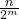with a relatively large power of two in the denominator. But as soon as we perform divisions, we leave the accurate world and round, usually in the way that the machine throws in front of our feet out of the box. But all other floating point operations can result in rounding as well. So rational numbers can be a way to go. So we can just naturally convert a double or float number into a rational number and continue working with that.

## Idea 2: Go for human readable fractions

Let us think of human readers. We are kind of comfortable with fractions like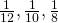and multiples of them, so basically every fraction that can be expressed with a denominator of 1, 2, 3, 4, 5, 6, 8, 10 or 12. The 12 is because of the omnipresence of the analog clock, the 10 because it is just one more decimal digit and the 8 because it is about the number of successive halving that we can still easily cope with. The idea would be to round to the nearest such fraction and to prefer the lower denominator when two adjacent values are equally far away. This works out well, because our set of allowed fractions between 0 and 1 is just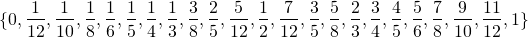and it is more or less trivial to program such a rounding algorithm, by just hard coding the limits, normalizing to the interval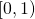and finding the right slot by binary search, for example. This is what we usually want to make numbers human readable and understandable. If we want more accuracy we can often just use the trick of going to % or or just shifting units by multiples of 1000, depending on what we are measuring or counting. This is often a bit better than just decimal numbers, and we can more often solve the issue of rounding with sum when some of the values we want to round are the same and just won’t come out the same of our rounding procedure.

## Idea 3: Use continuous fractions

With continuous fractions it is possible to express any real number in the form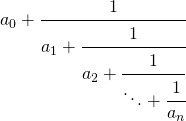or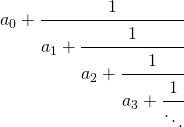For integers this is trivial, just use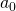. For negative numbers we just take the negative of the continuous fraction of the absolute value, so we can assume a non-integral positive number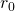.
This allows determiningby just taking the integer part of it.
Then we continue withand so on. Either we end up with an integer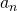at some point, which is the case for rational numbers or the continuous fraction continues infinitely, which is the case for irrational numbers. Now taking the continuous fraction just up to the first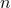elements, as in the first form and ignoring what comes after that, can in turn be converted into a rational number, by just doing some trivial rational arithmetic. It turns out, that this is a very good approximation for that size of the denominator. The funny thing is that this works even for irrational numbers under certain conditions. For example could we assume that we are dealing with numbers of the form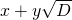for some fixed rational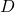and rational numbers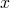and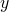. It is relatively easy to approximate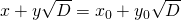with the largest integerthat is less or equal. Now we can calculate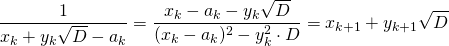and thus use this algorithm as another way to calculate rational approximations of square roots. In this case the continuous fraction becomes periodic and there is a surprising lot of interesting mathematics behind it, if you like to dig deeper.